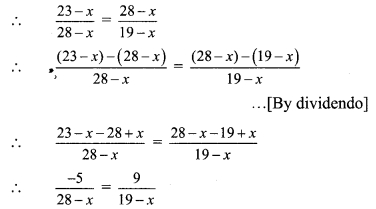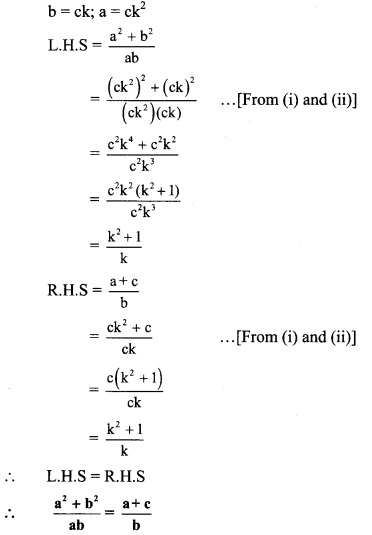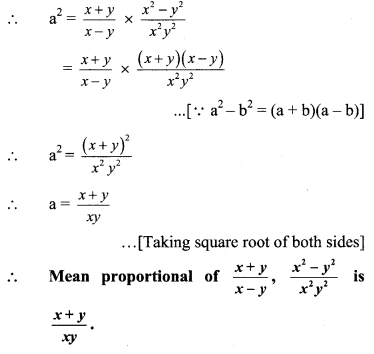# Maharashtra Board 9th Class Maths Part 1 Practice Set 4.5 Solutions Chapter 4 Ratio and Proportion

Balbharti Maharashtra State Board Class 9 Maths Solutions covers the Practice Set 4.5 Algebra 9th Class Maths Part 1 Answers Solutions Chapter 4 Ratio and Proportion.

## Practice Set 4.5 Algebra 9th Std Maths Part 1 Answers Chapter 4 Ratio and Proportion

Question 1.
Which number should be subtracted from 12, 16 and 21 so that resultant numbers are in continued proportion?
Solution:
Let the number to be subtracted be x.
∴ (12 – x), (16 – x) and (21 – x) are in continued proportion.∴ 84 – 4x = 80 – 5x
∴ 5x – 4x = 80 – 84
∴ x = -4
∴ -4 should be subtracted from 12,16 and 21 so that the resultant numbers in continued proportion.

Question 2.
If (28 – x) is the mean proportional of (23 – x) and (19 – x), then find the value ofx.
Solution:
(28 – x) is the mean proportional of (23 – x) and (19-x). …[Given]∴ -5(19 – x) = 9(28 – x)
∴ -95 + 5x = 252 – 9x
∴ 5x + 9x = 252 + 95
∴ 14x = 347
∴ x = $$\frac { 347 }{ 14 }$$

Question 3.
Three numbers are in continued proportion, whose mean proportional is 12 and the sum of the remaining two numbers is 26, then find these numbers.
Solution:
Let the first number be x.
∴ Third number = 26 – x
12 is the mean proportional of x and (26 – x).
∴ $$\frac { x }{ 12 }$$ = $$\frac { 12 }{ 26 – x }$$
∴ x(26 – x) = 12 x 12
∴ 26x – x2 = 144
∴ x2 – 26x + 144 = 0
∴ x2 – 18x – 8x + 144 = 0
∴ x(x – 18) – 8(x – 18) = 0
∴ (x – 18) (x – 8) = 0
∴ x = 18 or x = 8
∴ Third number = 26 – x = 26 – 18 = 8 or 26 – x = 26 – 8 = 18
∴ The numbers are 18, 12, 8 or 8, 12, 18.

Question 4.
If (a + b + c)(a – b + c) = a2 + b2 + c2, show that a, b, c are in continued proportion.
Solution:
(a + b + c)(a – b + c) = a2 + b2 + c2 …[Given]
∴ a(a – b + c) + b(a – b + c) + c(a – b + c) = a2 + b2 + c2
∴ a2 – ab + ac + ab – b2 + be + ac – be + c2 = a2 + b2 + c2
∴ a2 + 2ac – b2 + c2 = a2 + b2 + c2
∴ 2ac – b2 = b2
∴ 2ac = 2b2
∴ ac = b2
∴ b2 = ac
∴ a, b, c are in continued proportion.

Question 5.
If $$\frac { a }{ b }$$ = $$\frac { b }{ c }$$ and a, b, c > 0, then show that,
i. (a + b + c)(b – c) = ab – c2
ii. (a2 + b2)(b2 + c2) = (ab + be)2
iii. $$\frac{a^{2}+b^{2}}{a b}=\frac{a+c}{b}$$
Solution:
Let $$\frac { a }{ b }$$ = $$\frac { b }{ c }$$ = k
∴ b = ck
∴ a = bk =(ck)k
∴ a = ck2 …(ii)

i. (a + b + c)(b – c) = ab – c2
L.H.S = (a + b + c) (b – c)
= [ck2 + ck + c] [ck – c] … [From (i) and (ii)]
= c(k2 + k + 1) c (k – 1)
= c2 (k2 + k + 1) (k – 1)
R.H.S = ab – c2
= (ck2) (ck) – c2 … [From (i) and (ii)]
= c2k3 – c2
= c2(k3 – 1)
= c2 (k – 1) (k2 + k + 1) … [a3 – b3 = (a – b) (a2 + ab + b2]
∴ L.H.S = R.H.S
∴ (a + b + c) (b – c) = ab – c2

ii. (a2 + b2)(b2 + c2) = (ab + bc)2
b = ck; a = ck2
L.H.S = (a2 + b2) (b2 + c2)
= [(ck2) + (ck)2] [(ck)2 + c2] … [From (i) and (ii)]
= [c2k4 + c2k2] [c2k2 + c2]
= c2k2 (k2 + 1) c2 (k2 + 1)
= c4k2 (k2 + 1)2
R.H.S = (ab + bc)2
= [(ck2) (ck) + (ck)c]2 …[From (i) and (ii)]
= [c2k3 + c2k]2
= [c2k (k2 + 1)]2 = c4(k2 + 1)2
∴ L.H.S = R.H.S
∴ (a2 + b2) (b2 + c2) = (ab + bc)2

iii. $$\frac{a^{2}+b^{2}}{a b}=\frac{a+c}{b}$$9th Standard Algebra Practice Set 4.5 Question 6. Find mean proportional of $$\frac{x+y}{x-y}, \frac{x^{2}-y^{2}}{x^{2} y^{2}}$$.
Solution:
Let a be the mean proportional of $$\frac{x+y}{x-y}$$ and $$\frac{x^{2}-y^{2}}{x^{2} y^{2}}$$